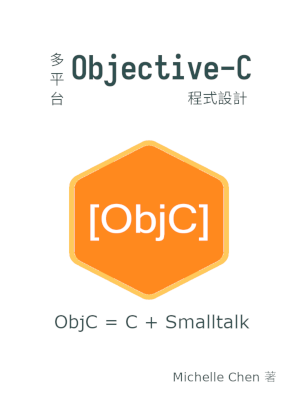#[Objective-C] 程式設計教學：控制物件的存取權限以實現封裝

## 陣列類別 `Array` 的公開界面宣告

``````/* array.h */
#pragma once

#import <Foundation/Foundation.h>
#include <stdbool.h>
#include <stddef.h>

typedef struct array_data_t array_data_t;

@interface Array : NSObject {
array_data_t *data;
}

-(Array *) init;
-(void) dealloc;
-(size_t) size;
-(id) at: (size_t) index;
-(bool) push: (id) obj;
@end``````

## 陣列類別 `Array` 的內部實作

``````struct array_data_t {
size_t size;
size_t capacity;
size_t tail;
id *elems;
};``````

``````@interface Array ()
-(bool) expand;
@end``````

``````-(Array *) init
{
if (!self)
return self;

self = [super init];

data = \
(array_data_t *) malloc(sizeof(array_data_t));
if (!data) {
[self release];
self = nil;
return self;
}

data->size = 0;
data->capacity = 2;
data->tail = 0;

data->elems = \
(id *) malloc(data->capacity * sizeof(id));
if (!(data->elems)) {
free(data);
[self release];
self = nil;
return self;
}

return self;
}``````

``````-(void) dealloc
{
if (!self)
return;

if (!data) {
[super dealloc];
return;
}

for (size_t i = 0; i < data->size; ++i) {
size_t index = (i + data->head) % data->size;
if (nil != data->elems[index])
[data->elems[index] release];
}

free(data->elems);
free(data);

[super dealloc];
}``````

``````-(bool) push: (id)obj
{
if (![self expand])
return false;

if (data->size > 0)
data->tail = (data->tail + 1) % data->capacity;

data->elems[data->tail] = obj;
data->size += 1;

return true;
}``````

``````/* array.m */
#include <stdbool.h>
#include <stdlib.h>
#import "array.h"

/* Private fields. */
struct array_data_t {
size_t size;
size_t capacity;
size_t tail;
id *elems;
};

/* Private messages. */
@interface Array ()
- (bool) expand;
@end

@implementation Array
-(Array *) init
{
if (!self)
return self;

self = [super init];

data = (array_data_t *) malloc(sizeof(array_data_t));
if (!data) {
[self release];
self = nil;
return self;
}

data->size = 0;
data->capacity = 2;
data->tail = 0;

data->elems = (id *) malloc(data->capacity * sizeof(id));
if (!(data->elems)) {
free(data);
[self release];
self = nil;
return self;
}

for (size_t i = 0; i < data->capacity; ++i)
data->elems[i] = NULL;

return self;
}

-(void) dealloc
{
if (!self)
return;

if (!data) {
[super dealloc];
return;
}

for (size_t i = 0; i < data->size; ++i) {
size_t index = (i + data->head) % data->size;
if (nil != data->elems[index])
[data->elems[index] release];
}

free(data->elems);
free(data);

[super dealloc];
}

-(size_t) size
{
return data->size;
}

-(id) at: (size_t)index
{
NSAssert(index < data->size, @"Invalid index\n");

size_t i = (index + data->head) % data->size;

return data->elems[i];
}

-(bool) push: (id) obj
{
if (![self expand])
return false;

if (data->size > 0)
data->tail = (data->tail + 1) % data->capacity;

data->elems[data->tail] = obj;
data->size += 1;

return true;
}

-(bool) expand
{
if (data->size < data->capacity)
return true;

data->capacity <<= 1;
id *old_elems = data->elems;
id *new_elems = (id *) malloc(data->capacity * sizeof(id));
if (!new_elems)
return false;

size_t j = 0;
size_t sz = 0;
while (sz < data->size) {
new_elems[j] = old_elems[i];

i = (i + 1) % data->size;
j = (j + 1) % data->capacity;
sz++;
}

data->tail = data->size - 1;

data->elems = new_elems;
free(old_elems);

return true;
}
@end``````

## 使用該類別的外部程式

``````/* main.m */
#import <Foundation/Foundation.h>
#include <stdio.h>
#import "array.h"

int main(void)
{
NSAutoreleasePool *pool = \
[[NSAutoreleasePool alloc] init];
if (!pool)
return 1;

Array *arr = nil;

arr = [[[Array alloc] init] autorelease];
if (!arr) {
perror("Failed to allocate arr\n");
goto ERROR;
}

NSArray *data = [NSArray arrayWithObjects:
[NSNumber numberWithInt: 3],
[NSNumber numberWithInt: 4],
[NSNumber numberWithInt: 5],
[NSNumber numberWithInt: 6],
[NSNumber numberWithInt: 7],
nil
];
for (id obj in data) {
if (![arr push: obj]) {
perror("Failed to push data\n");
goto ERROR;
}
}

for (size_t i = 0; i < [arr size]; i++) {
int a = [[arr at:i] intValue];
int b = [[data objectAtIndex: i] intValue];
if (!(a == b)) {
fprintf(stderr, "Unequal value: %d %d\n", a, b);
goto ERROR;
}
}

[pool drain];

return 0;

ERROR:
[pool drain];

return 1;
}``````

## 編譯此範例程式

``\$ clang -o array array.m main.m -lobjc -framework Foundation``

``\$ gcc -std=c11  -o array array.m main.m -lobjc -lgnustep-base -I /usr/include/GNUstep -L /usr/lib/GNUstep -fconstant-string-class=NSConstantString``

``\$ clang -std=c11 -o arrayDemo array.m main.m -lobjc -lgnustep-base -I /usr/include/GNUstep -I /usr/lib/gcc/x86_64-linux-gnu/7/include -L /usr/lib/GNUstep -fconstant-string-class=NSConstantString``

## 繼續深入